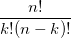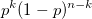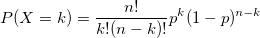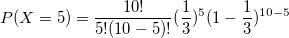# Binomial Random Variables

• Difficulty Level : Medium
• Last Updated : 30 Nov, 2021

In this post, we’ll discuss Binomial Random Variables.
Prerequisite : Random Variables
A specific type of discrete random variable that counts how often a particular event occurs in a fixed number of tries or trials.
For a variable to be a binomial random variable, ALL of the following conditions must be met:

1. There are a fixed number of trials (a fixed sample size).
2. On each trial, the event of interest either occurs or does not.
3. The probability of occurrence (or not) is the same on each trial.
4. Trials are independent of one another.

Mathematical Notations

```n = number of trials
p = probability of success in each trial
k = number of success in n trials```

Now we try to find out the probability of k success in n trials.
Here the probability of success in each trial is p independent of other trials.
So we first choose k trials in which there will be a success and in rest n-k trials there will be a failure. Number of ways to do so isSince all n events are independent, hence the probability of k success in n trials is equivalent to multiplication of probability for each trial.
Here its k success and n-k failures, So probability for each way to achieve k success and n-k failure isHence final probability is

```(number of ways to achieve k success
and n-k failures)
*
(probability for each way to achieve k
success and n-k failure)```

Then Binomial Random Variable Probability is given by:Let X be a binomial random variable with the number of trials n and probability of success in each trial be p.
Expected number of success is given by

`E[X] = np`

Variance of number of success is given by

`Var[X] = np(1-p)`

Example 1 : Consider a random experiment in which a biased coin (probability of head = 1/3) is thrown for 10 times. Find the probability that the number of heads appearing will be 5.
Solution :

```Let X be binomial random variable
with n = 10 and p = 1/3
P(X=5) = ?```` `` `

Here is the implementation for the same

## C++

 `// C++ program to compute Binomial Probability``#include ``#include ``using` `namespace` `std;`` ` `// function to calculate nCr i.e., number of ``// ways to choose r out of n objects``int` `nCr(``int` `n, ``int` `r)``{``    ``// Since nCr is same as nC(n-r)``    ``// To decrease number of iterations``    ``if` `(r > n / 2)``        ``r = n - r;`` ` `    ``int` `answer = 1;``    ``for` `(``int` `i = 1; i <= r; i++) {``        ``answer *= (n - r + i);``        ``answer /= i;``    ``}`` ` `    ``return` `answer;``}`` ` `// function to calculate binomial r.v. probability``float` `binomialProbability(``int` `n, ``int` `k, ``float` `p)``{``    ``return` `nCr(n, k) * ``pow``(p, k) *``                ``pow``(1 - p, n - k);``}`` ` `// Driver code``int` `main()``{`` ` `    ``int` `n = 10;``    ``int` `k = 5;``    ``float` `p = 1.0 / 3;`` ` `    ``float` `probability = binomialProbability(n, k, p);`` ` `    ``cout << ``"Probability of "` `<< k;``    ``cout << ``" heads when a coin is tossed "` `<< n;``    ``cout << ``" times where probability of each head is "` `<< p << endl;``    ``cout << ``" is = "` `<< probability << endl;``}`

## Java

 `// Java program to compute Binomial Probability`` ` `import` `java.util.*;`` ` `class` `GFG``{``    ``// function to calculate nCr i.e., number of ``    ``// ways to choose r out of n objects``    ``static` `int` `nCr(``int` `n, ``int` `r)``    ``{``        ``// Since nCr is same as nC(n-r)``        ``// To decrease number of iterations``        ``if` `(r > n / ``2``)``            ``r = n - r;``     ` `        ``int` `answer = ``1``;``        ``for` `(``int` `i = ``1``; i <= r; i++) {``            ``answer *= (n - r + i);``            ``answer /= i;``        ``}``     ` `        ``return` `answer;``    ``}``     ` `    ``// function to calculate binomial r.v. probability``    ``static` `float` `binomialProbability(``int` `n, ``int` `k, ``float` `p)``    ``{``        ``return` `nCr(n, k) * (``float``)Math.pow(p, k) * ``                        ``(``float``)Math.pow(``1` `- p, n - k);``    ``}``     ` `    ``// Driver code``    ``public` `static` `void` `main(String[] args)``    ``{``        ``int` `n = ``10``;``        ``int` `k = ``5``;``        ``float` `p = (``float``)``1.0` `/ ``3``;``     ` `        ``float` `probability = binomialProbability(n, k, p);``         ` `        ``System.out.print(``"Probability of "` `+k);``        ``System.out.print(``" heads when a coin is tossed "` `+n);``        ``System.out.println(``" times where probability of each head is "` `+p);``        ``System.out.println( ``" is = "` `+ probability );``    ``}``}`` ` `/* This code is contributed by Mr. Somesh Awasthi */`

## Python3

 `# Python3 program to compute Binomial ``# Probability`` ` `# function to calculate nCr i.e.,``# number of ways to choose r out``# of n objects``def` `nCr(n, r):``     ` `    ``# Since nCr is same as nC(n-r)``    ``# To decrease number of iterations``    ``if` `(r > n ``/` `2``):``        ``r ``=` `n ``-` `r;`` ` `    ``answer ``=` `1``;``    ``for` `i ``in` `range``(``1``, r ``+` `1``):``        ``answer ``*``=` `(n ``-` `r ``+` `i);``        ``answer ``/``=` `i;`` ` `    ``return` `answer;`` ` `# function to calculate binomial r.v.``# probability``def` `binomialProbability(n, k, p):`` ` `    ``return` `(nCr(n, k) ``*` `pow``(p, k) ``*` `                        ``pow``(``1` `-` `p, n ``-` `k));`` ` `# Driver code``n ``=` `10``;``k ``=` `5``;``p ``=` `1.0` `/` `3``;`` ` `probability ``=` `binomialProbability(n, k, p);`` ` `print``(``"Probability of"``, k, ``      ``"heads when a coin is tossed"``, end ``=` `" "``);``print``(n, ``"times where probability of each head is"``, ``                                      ``round``(p, ``6``));``print``(``"is = "``, ``round``(probability, ``6``));`` ` `# This code is contributed by mits`

## C#

 `// C# program to compute Binomial``// Probability.``using` `System;`` ` `class` `GFG {``     ` `    ``// function to calculate nCr``    ``// i.e., number of ways to ``    ``// choose r out of n objects``    ``static` `int` `nCr(``int` `n, ``int` `r)``    ``{``         ` `        ``// Since nCr is same as``        ``// nC(n-r) To decrease ``        ``// number of iterations``        ``if` `(r > n / 2)``            ``r = n - r;``     ` `        ``int` `answer = 1;``        ``for` `(``int` `i = 1; i <= r; i++)``        ``{``            ``answer *= (n - r + i);``            ``answer /= i;``        ``}``     ` `        ``return` `answer;``    ``}``     ` `    ``// function to calculate binomial``    ``// r.v. probability``    ``static` `float` `binomialProbability(``                  ``int` `n, ``int` `k, ``float` `p)``    ``{``        ``return` `nCr(n, k) * ``                   ``(``float``)Math.Pow(p, k)``                ``* (``float``)Math.Pow(1 - p,``                                 ``n - k);``    ``}``     ` `    ``// Driver code``    ``public` `static` `void` `Main()``    ``{``        ``int` `n = 10;``        ``int` `k = 5;``        ``float` `p = (``float``)1.0 / 3;``     ` `        ``float` `probability = ``              ``binomialProbability(n, k, p);``         ` `        ``Console.Write(``"Probability of "``                                      ``+ k);``        ``Console.Write(``" heads when a coin "``                       ``+ ``"is tossed "` `+ n);``        ``Console.Write(``" times where "``           ``+ ``"probability of each head is "``                                      ``+ p);``        ``Console.Write( ``" is = "``                           ``+ probability );``    ``}``}`` ` `// This code is contributed by nitin mittal.`

## PHP

 ` ``\$n` `/ 2)``        ``\$r` `= ``\$n` `- ``\$r``;`` ` `    ``\$answer` `= 1;``    ``for` `(``\$i` `= 1; ``\$i` `<= ``\$r``; ``\$i``++) {``        ``\$answer` `*= (``\$n` `- ``\$r` `+ ``\$i``);``        ``\$answer` `/= ``\$i``;``    ``}`` ` `    ``return` `\$answer``;``}`` ` `// function to calculate binomial r.v.``// probability``function` `binomialProbability(``\$n``, ``\$k``, ``\$p``)``{``    ``return` `nCr(``\$n``, ``\$k``) * pow(``\$p``, ``\$k``) *``                   ``pow(1 - ``\$p``, ``\$n` `- ``\$k``);``}`` ` `// Driver code``    ``\$n` `= 10;``    ``\$k` `= 5;``    ``\$p` `= 1.0 / 3;`` ` `    ``\$probability` `= ``         ``binomialProbability(``\$n``, ``\$k``, ``\$p``);`` ` `    ``echo` `"Probability of "` `. ``\$k``;``    ``echo` `" heads when a coin is tossed "``                                    ``. ``\$n``;``    ``echo` `" times where probability of "``                 ``. ``"each head is "` `. ``\$p` `;``    ``echo` `" is = "` `. ``\$probability` `;`` ` `// This code is contributed by nitin mittal.``?>`

## Javascript

 ``

Output:

```Probability of 5 heads when a coin is tossed 10 times where probability of each head is 0.333333
is = 0.136565```

Reference
stat200
This article is contributed by Pratik Chhajer . If you like GeeksforGeeks and would like to contribute, you can also write an article using write.geeksforgeeks.org or mail your article to review-team@geeksforgeeks.org. See your article appearing on the GeeksforGeeks main page and help other Geeks.